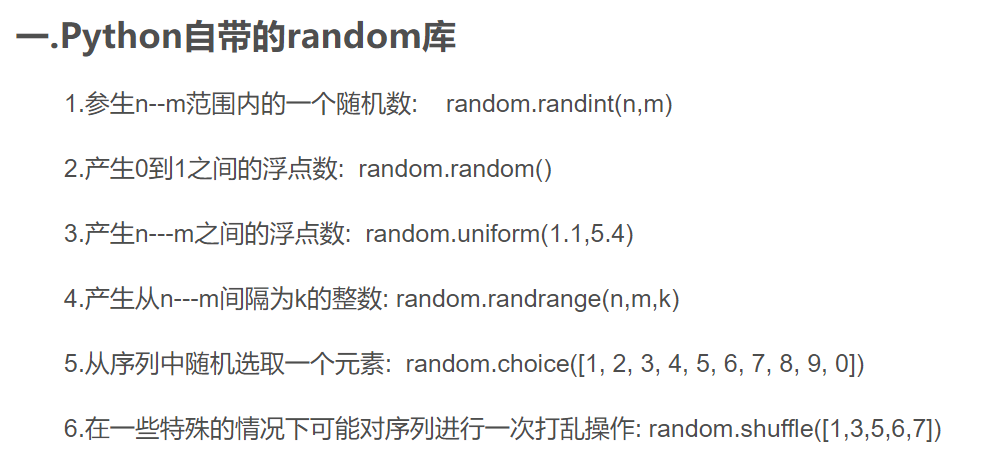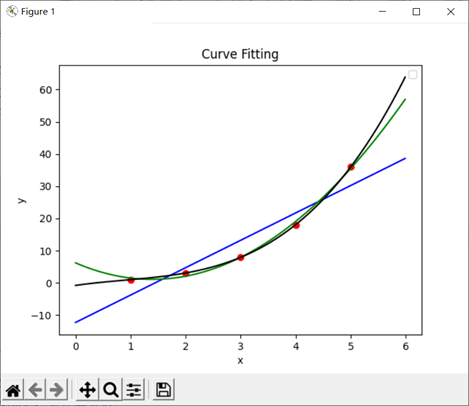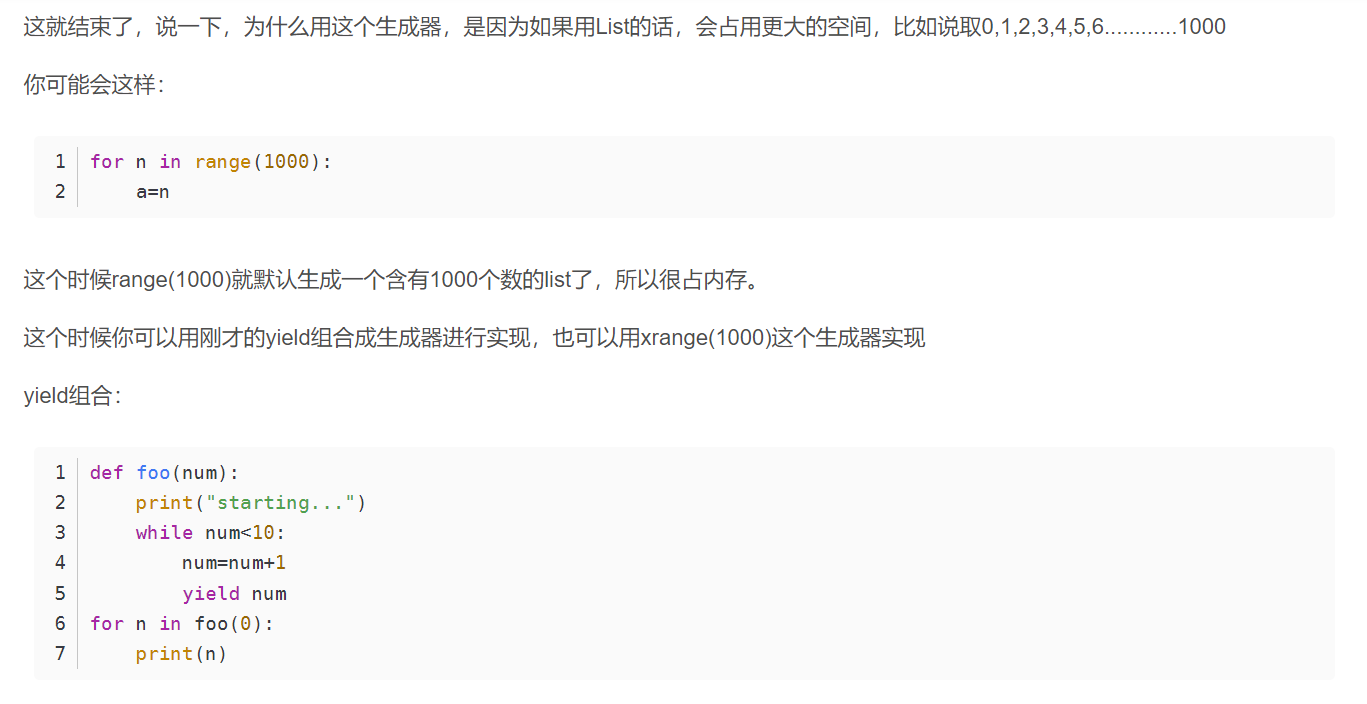# Python 学习笔记（附 Pytorch)

## 一、基础

可以强制转换：x=int(input()) （或者float)

for循环：

for i in A：                   # A是一个list，这个和C++里面的auto遍历很像
for i in range(a,b)        # 等价于，for(int i=a;i<b;++i)

while循环：

while i<n:
while 1:

print函数：

print(A,'2333',B,C) #可以输出很多个，之间用一个空格隔开
#每次print默认会输出换行符

print(...,end='') #强制规定最后的输出符号，这里就是最后什么也不输出
print(...,end=' ') #最后输出空格
print(...,end='\n') #最后输出换行符，这个和直接print(...)是一样的

ord(i) 这里i是一个字符，返回它的ascii值

len(A) 这里A是一个list，返回它的size

## 二、进阶

### 生成随机数：### list的一些操作

A=str(input())
A=A.split('char') #按照char字符把A这个字符串拆分成若干段，存在list里，返回这个list，重新赋值给A
A.append(x) #A的末尾加入一个元素x  （push_back)
A.insert(1,x) #在原下标为0和1之间插入一个元素x
A.pop(1) #删除下标为1位置的元素

### 文件输入输出操作

with open('1.txt','r') as f:   #打开文件，类型为只读 'w'为只写，'a'为文末追加只写，’w+'为可读可写
#如果什么都不加，默认是'r'

#还需要去掉\n处理

### 关于读入csv文件

https://www.cnblogs.com/qican/p/11122206.html

import csv
f=csv.reader(open('name.csv','r')) #读入csv文件

### 关于用曲线拟合散点，以及绘图

x0和y0是两个list，在没展示的代码里面进行了赋值

import pandas as pd
import numpy as np
import matplotlib.pyplot as plt
from scipy import optimize as op

x=np.array(x0)
y=np.array(y0)
plt.scatter(x,y,marker='o',color='red') #绘制原始数据的点
x_=np.arange(0,6,0.01) #以0.01为间隔，在[0,6]中取大量的点，以便绘制曲线

#绘制一次函数拟合曲线
f1=np.polyfit(x,y,1)#计算系数,f1是两个系数组成的list
f_1=np.poly1d(f1) #这里用poly1d，就转化成了一个多项式f_1
plt.plot(x_,f_1(x_),color='blue')

#绘制二次函数拟合曲线
f2=np.polyfit(x,y,2)#计算系数
f_2=np.poly1d(f2)
plt.plot(x_,f_2(x_),color='green')

#绘制三次函数拟合曲线
f3=np.polyfit(x,y,3)#计算系数
f_3=np.poly1d(f3)
plt.plot(x_,f_3(x_),color='black')

#添加表头等信息
print('红点是csv文件中的数据，蓝色是一次方程拟合，绿色是二次方程拟合，黑色是三次方程拟合：')
plt.xlabel('x') #绘制横坐标表头
plt.ylabel('y') #绘制纵坐标表头
plt.legend()
plt.title('Curve Fitting') #绘制标题
plt.show()### 关于yield和return

https://blog.csdn.net/mieleizhi0522/article/details/82142856摘自：https://blog.csdn.net/mieleizhi0522/article/details/82142856

## Pytorch

https://www.pytorch123.com/SecondSection/what_is_pytorch/

https://zhuanlan.zhihu.com/p/25572330

posted @ 2021-07-26 09:08  *Miracle*  阅读(87)  评论(0编辑  收藏  举报